author: 魔方 12139

1. 无法在编辑时创建和编辑 3D 模型，无直接导入通用的 3D 模型的方法。
2. 无法直接在房间编辑时在 3D 空间中放置物体。
3. 必须自己处理 3D 世界变换、投影变换等。
4. 必须自己处理 3D 空间中的碰撞判断。
5. 房间背景、前景设置以及图块使用可能出错。

## 改变投影方式

• 正交投影（orthogonal projection）

过点作一直线垂直于目标平面，垂足作为投影点。这也是“正交”一词的含义如图：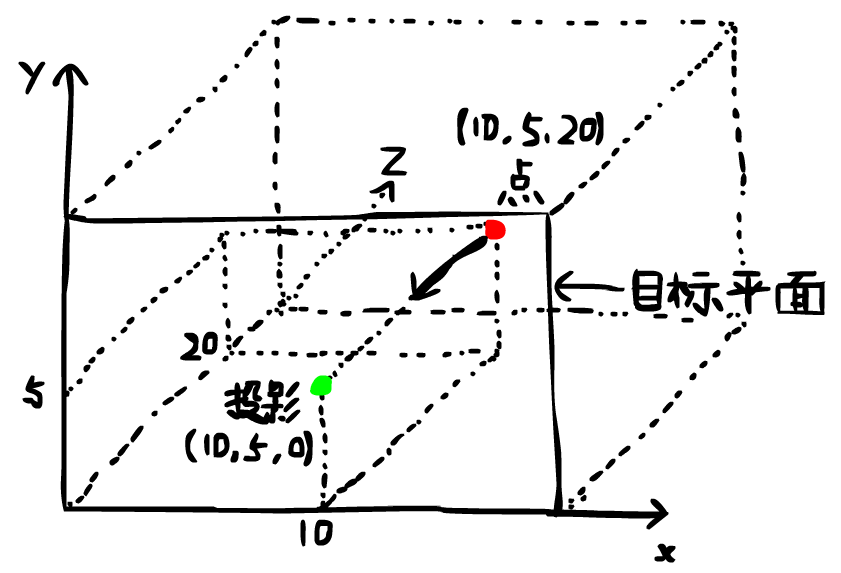• 透视投影（perspective projection）

将点与视点（也就是你从哪儿看这个空间）连线，与目标平面的交点作为投影点。如图：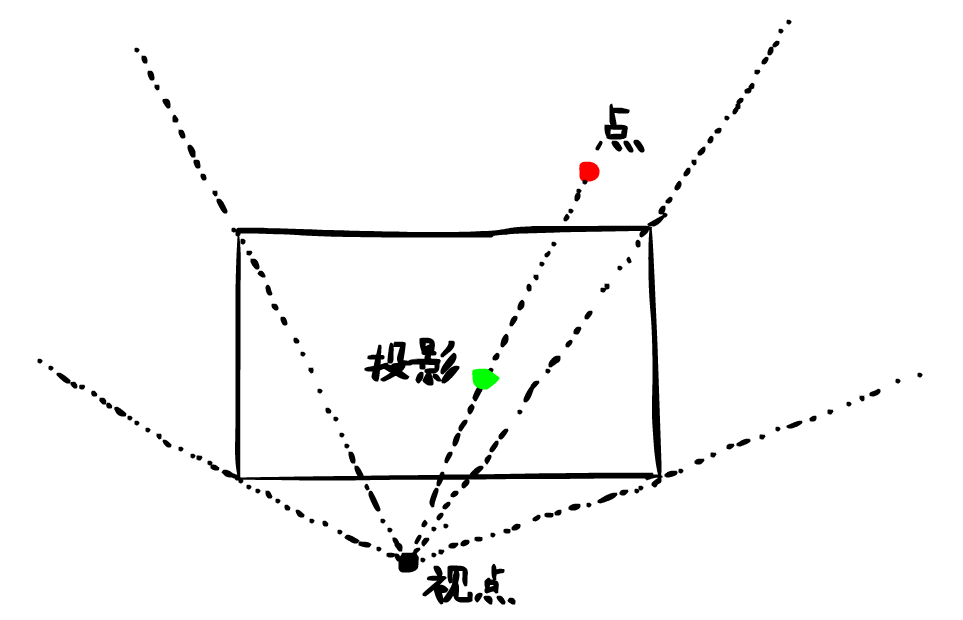这种投影方式产生的效果和人眼的投影效果相近。

GM8 中，设置这两种投影方式的函数分别如下：

• `d3d_set_projection_ortho(x, y, w, h, angle)` 设置正交投影。参数 x 是被投影部分的左上角水平坐标，参数 y 是被投影部分的左上角竖直坐标，参数 w 是被投影部分的宽度，参数 h 是被投影部分的高度，参数 angle 是被投影部分的旋转角度。
• `d3d_set_projection_perspective(x, y, w, h, angle)` 设置透视投影。参数同上。

```1 d3d_set_projection_perspective(0, 0, room_width, room_height, 0); ```

## 绘制 3D 图形

```1 2 3 4 5 draw_primitive_begin(kind); draw_vertex(x, y); draw_vertex(x, y); ... draw_primitive_end(); ```

```1 2 3 4 5 d3d_primitive_begin(kind); d3d_vertex(x, y, z); d3d_vertex(x, y, z); ... d3d_primitive_end(); ```

```1 2 3 4 5 6 7 8 9 10 11 12 13 14 15 16 17 18 19 20 21 22 23 24 25 26 27 28 29 30 31 32 33 34 35 36 37 38 // 设置透视投影方式 d3d_set_projection_perspective(0, 0, room_width, room_height, 0); // 拓扑结构：线列表（忘了的去复习） d3d_primitive_begin(pr_linelist); // 顶面 d3d_vertex(0, 0, 0); d3d_vertex(100, 0, 0); d3d_vertex(100, 0, 0); d3d_vertex(100, 100, 0); d3d_vertex(100, 100, 0); d3d_vertex(0, 100, 0); d3d_vertex(0, 100, 0); d3d_vertex(0, 0, 0); // 侧面 d3d_vertex(0, 0, 0); d3d_vertex(0, 0, 100); d3d_vertex(100, 0, 0); d3d_vertex(100, 0, 100); d3d_vertex(100, 100, 0); d3d_vertex(100, 100, 100); d3d_vertex(0, 100, 0); d3d_vertex(0, 100, 100); // 底面 d3d_vertex(0, 0, 100); d3d_vertex(100, 0, 100); d3d_vertex(100, 0, 100); d3d_vertex(100, 100, 100); d3d_vertex(100, 100, 100); d3d_vertex(0, 100, 100); d3d_vertex(0, 100, 100); d3d_vertex(0, 0, 100); d3d_primitive_end(); // 把投影方式设置回去 d3d_set_projection_ortho(0, 0, room_width, room_height, 0); ```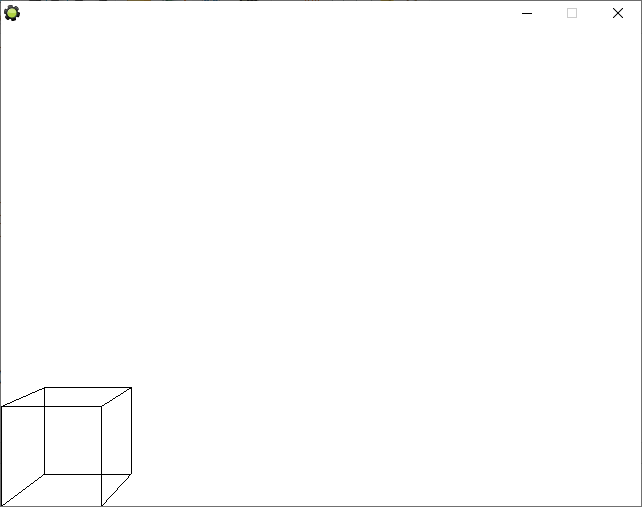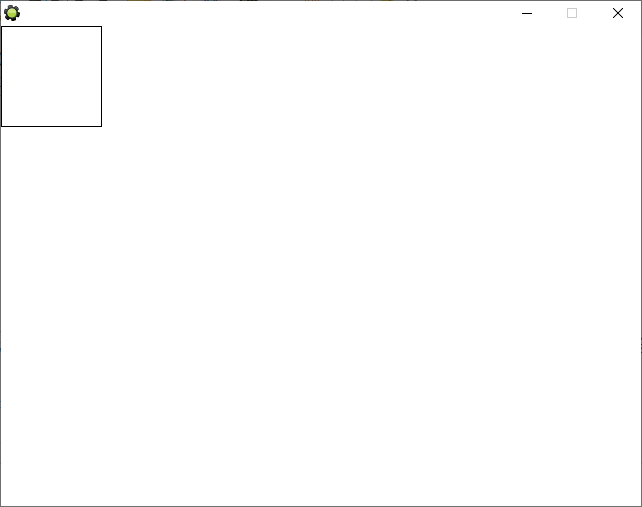```1 2 3 4 5 6 7 8 9 10 11 d3d_vertex_color(x, y, z, col, alpha); d3d_vertex_normal(x, y, z, nx, ny, nz); d3d_vertex_normal_color(x, y, z, nx, ny, nz); d3d_vertex_normal_texture(x, y, z, nx, ny, nz, xtex, ytex); d3d_vertex_color(x, y, z, col, alpha); d3d_vertex_normal(x, y, z, nx, ny, nz); d3d_vertex_normal_color(x, y, z, nx, ny, nz, col, alpha); d3d_vertex_normal_texture(x, y, z, nx, ny, nz, xtex, ytex); d3d_vertex_normal_texture_color(x, y, z, nx, ny, nz, xtex, ytex, col, alpha); d3d_vertex_texture(x, y, z, xtex, ytex); d3d_vertex_texture_color(x, y, z, xtex, ytex, col, alpha); ```

```1 2 3 4 5 6 d3d_draw_block(x1, y1, z1, x2, y2, z2, texid, hrepeat, vrepeat); d3d_draw_cylinder(x1, y1, z1, x2, y2, z2, texid, hrepeat, vrepeat, closed, steps); d3d_draw_cone(x1, y1, z1, x2, y2, z2, texid, hrepeat, vrepeat, closed, steps); d3d_draw_ellipsoid(x1, y1, z1, x2, y2, z2, texid, hrepeat, vrepeat, steps); d3d_draw_wall(x1, y1, z1, x2, y2, z2, texid, hrepeat, vrepeat); d3d_draw_floor(x1, y1, z1, x2, y2, z2, texid, hrepeat, vrepeat); ```

## 设置 3D 变换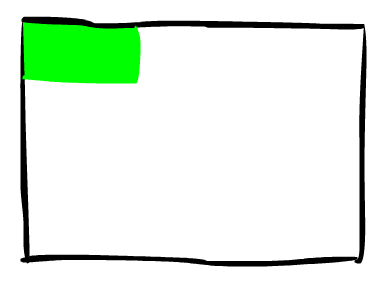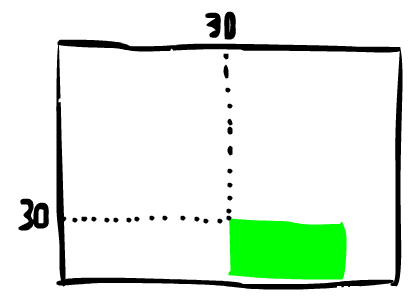• `d3d_transform_set_identity()` 设置当前变换为单位变换，也就是什么都不变换。
• `d3d_transform_set_translation(xt, yt, zt)` 设置当前变换为到指定点的平移变换。
• `d3d_transform_set_scaling(xs, ys, zs)` 设置当前变换为指定尺寸的伸缩变换。
• `d3d_transform_set_rotation_x(angle)` 设置当前变换为指定角度的绕 x 轴旋转变换。
• `d3d_transform_set_rotation_y(angle)` 设置当前变换为指定角度的绕 y 轴旋转变换。
• `d3d_transform_set_rotation_z(angle)` 设置当前变换为指定角度的绕 z 轴旋转变换。
• `d3d_transform_set_rotation_axis(xa, ya, za, angle)` 设置当前变换为绕指定方向旋转指定角度的旋转变换。
• `d3d_transform_add_translation(xt, yt, zt)` 在当前变换上追加到指定点的平移变换。
• `d3d_transform_add_scaling(xs, ys, zs)` 在当前变换上追加指定尺寸的伸缩变换。
• `d3d_transform_add_rotation_x(angle)` 在当前变换上追加指定角度的绕 x 轴旋转变换。
• `d3d_transform_add_rotation_y(angle)` 在当前变换上追加指定角度的绕 y 轴旋转变换。
• `d3d_transform_add_rotation_z(angle)` 在当前变换上追加指定角度的绕 z 轴旋转变换。
• `d3d_transform_add_rotation_axis(xa, ya, za, angle)` 在当前变换上追加绕指定方向旋转指定角度的旋转变换。

```1 2 3 rotation_x = 0; rotation_y = 0; rotation_z = 0; ```

```1 2 3 rotation_x += rotation_x_speed; rotation_y += rotation_y_speed; rotation_z += rotation_z_speed; ```

```1 2 3 4 5 6 7 8 9 10 11 12 13 14 15 16 17 18 19 20 21 22 23 24 25 26 27 28 29 30 31 32 33 34 35 36 37 38 39 40 d3d_set_projection_perspective(0, 0, room_width, room_height, 0); d3d_transform_set_scaling(scaling_x, scaling_y, scaling_z); d3d_transform_add_rotation_x(rotation_x); d3d_transform_add_rotation_y(rotation_y); d3d_transform_add_rotation_z(rotation_z); d3d_transform_add_translation(x_3d, y_3d, z_3d); d3d_primitive_begin(pr_linelist); d3d_vertex(-50, -50, -50); d3d_vertex( 50, -50, -50); d3d_vertex( 50, -50, -50); d3d_vertex( 50, 50, -50); d3d_vertex( 50, 50, -50); d3d_vertex(-50, 50, -50); d3d_vertex(-50, 50, -50); d3d_vertex(-50, -50, -50); d3d_vertex(-50, -50, -50); d3d_vertex(-50, -50, 50); d3d_vertex( 50, -50, -50); d3d_vertex( 50, -50, 50); d3d_vertex( 50, 50, -50); d3d_vertex( 50, 50, 50); d3d_vertex(-50, 50, -50); d3d_vertex(-50, 50, 50); d3d_vertex(-50, -50, 50); d3d_vertex( 50, -50, 50); d3d_vertex( 50, -50, 50); d3d_vertex( 50, 50, 50); d3d_vertex( 50, 50, 50); d3d_vertex(-50, 50, 50); d3d_vertex(-50, 50, 50); d3d_vertex(-50, -50, 50); d3d_primitive_end(); d3d_set_projection_ortho(0, 0, room_width, room_height, 0); ```

```1 2 3 4 5 6 7 8 9 x_3d = 100; y_3d = 100; z_3d = 0; scaling_x = 1; scaling_y = 1; scaling_z = 1; rotation_x_speed = 3; rotation_y_speed = 0; rotation_z_speed = 0; ```

```1 2 3 4 5 6 7 8 9 x_3d = 100; y_3d = 200; z_3d = 100; scaling_x = 1; scaling_y = 1; scaling_z = 1; rotation_x_speed = 0; rotation_y_speed = 3; rotation_z_speed = 0; ```

```1 2 3 4 5 6 7 8 9 x_3d = 300; y_3d = 100; z_3d = 0; scaling_x = 2; scaling_y = 1; scaling_z = 1; rotation_x_speed = 0; rotation_y_speed = 0; rotation_z_speed = 3; ```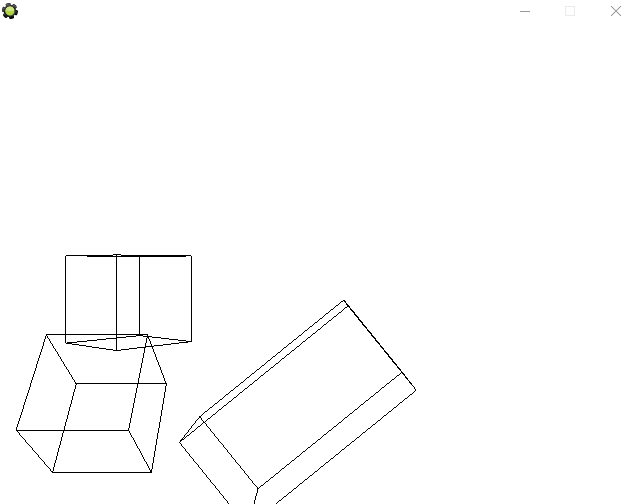## 3D 绘制与 2D 绘制的冲突```1 2 3 d3d_set_projection_perspective(0, 0, room_width, room_height, 0); draw_sprite(sprite0, 0, 100, 100); ```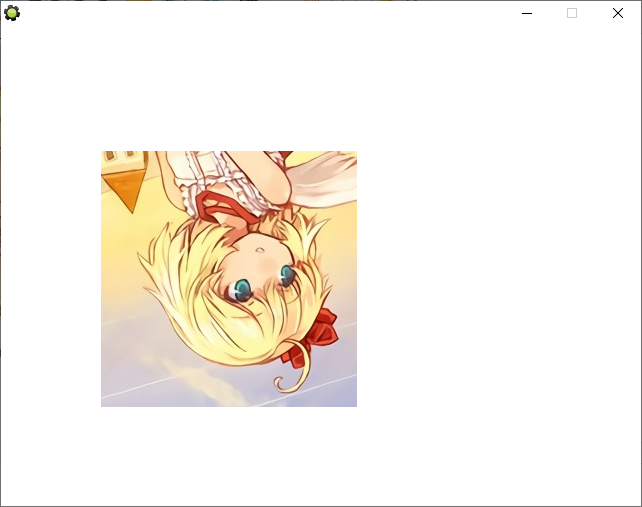• 左上(0, 0) -> (100, 100)
• 左下(0, 1) -> (100, 356)
• 右上(1, 0) -> (356, 100)
• 右下(1, 1) -> (356, 356)

## 其他 3D 功能

GM8 提供的 3D 功能还包括以下方面：

1. 创建、保存和读取 3D 模型（完全使用代码，无可视性，且保存和读取的格式都仅限 GM 独家）
2. 设置灯光和雾（没怎么用过）
3. 暂存和读取变换方式（也就是说可以把当前的变换方式保存起来，以后要用的时候读出来即可。通过栈数据结构实现）

## GMS(2) 的 3D 功能

GMS1 中为了兼容，提供了和 GM8 的 d3d_系列函数相同的函数，但顶点缓冲区（Vertex Buffer），矩阵（Matrix）和 gpu_系列函数等更接近底层的功能已经出现；到了 GMS2，d3d_系列函数已被完全删除，3D 绘制功能都需要通过上述的三方面来实现。不过就我看来，只要弄懂了 3D 绘制的本质，GM8 和 GMS(2) 也没有什么难以理解的差别。